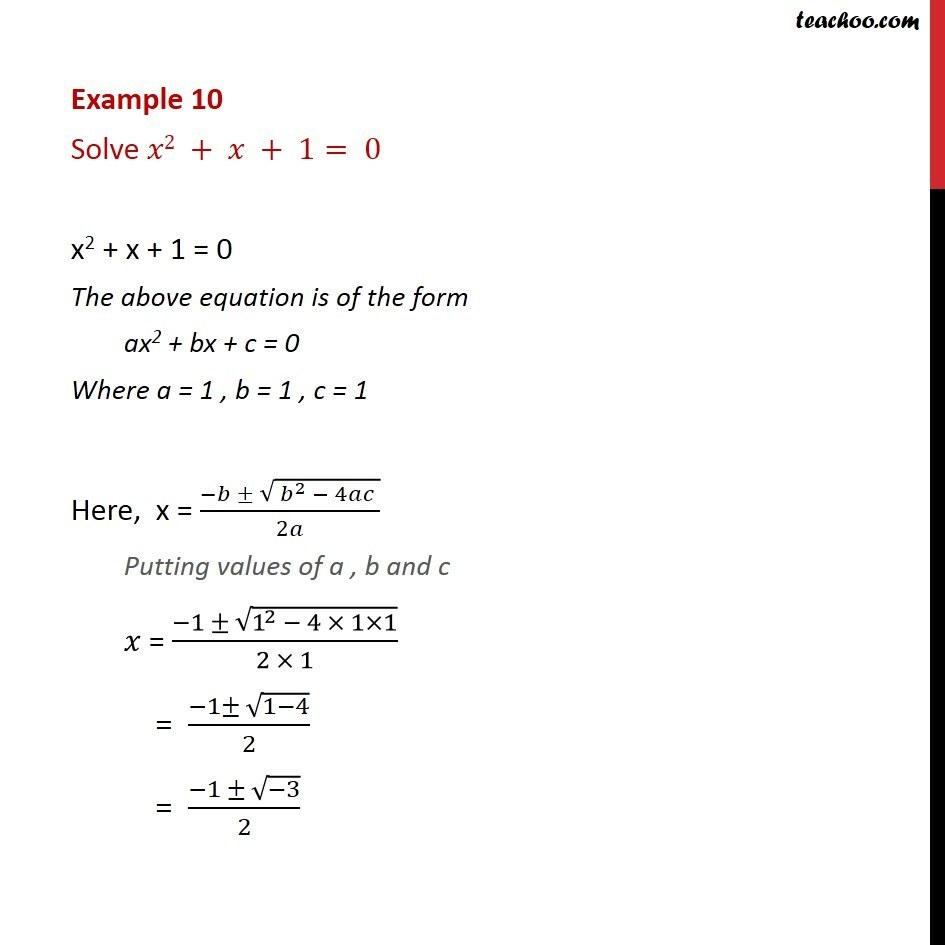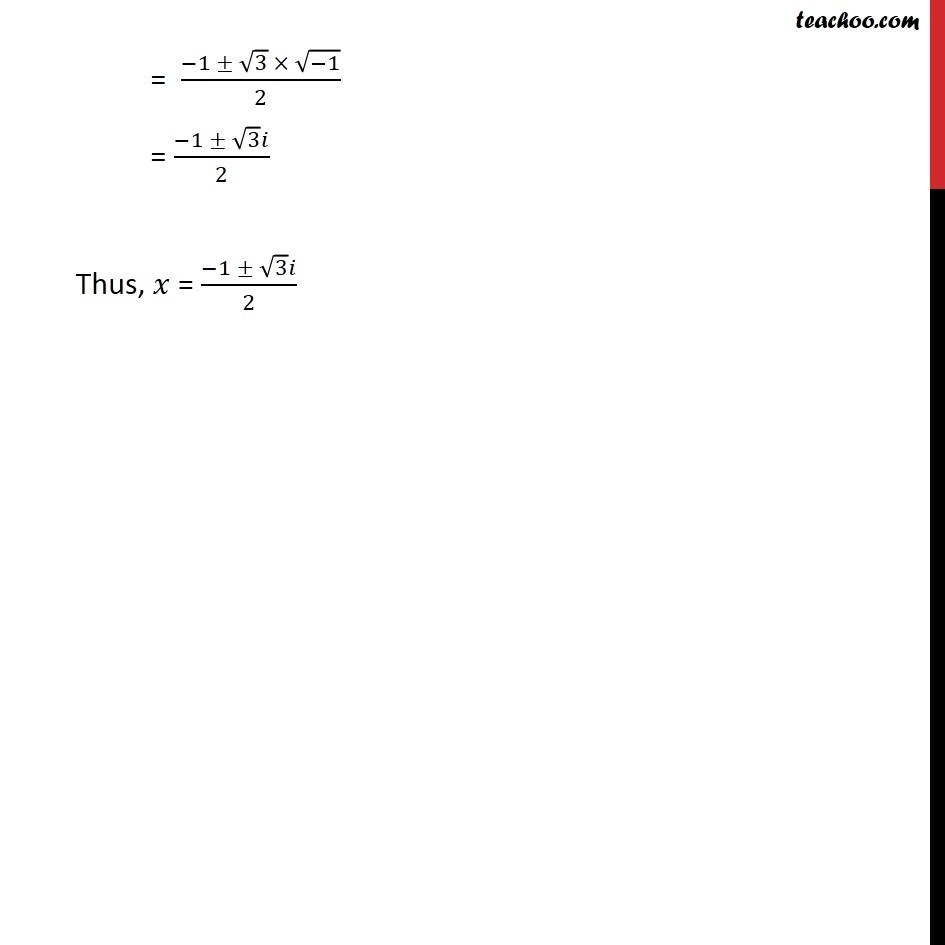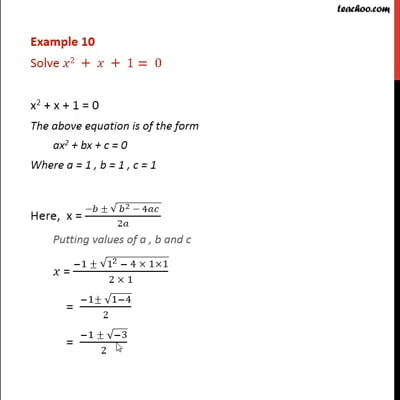Chapter 4 Class 11 Complex Numbers
Concept wiseThis video is only available for Teachoo black users

Learn in your speed, with individual attention - Teachoo Maths 1-on-1 Class

### Transcript

Question 4 Solve 2 + + 1= 0 x2 + x + 1 = 0 The above equation is of the form ax2 + bx + c = 0 Where a = 1 , b = 1 , c = 1 Here, x = ( ( ^2 4 ))/2 Putting values of a , b and c = ( 1 (1^2 4 1 1))/(2 1) = ( 1 (1 4))/2 = ( 1 ( 3))/2 = ( 1 3 ( 1))/2 = ( 1 3 )/2 Thus, = ( 1 3 )/2